# Class 10 Science: CBSE Sample Question Paper- Term II (2021-22) - 1 Notes | Study CBSE Sample Papers For Class 10 - Class 10

## Class 10: Class 10 Science: CBSE Sample Question Paper- Term II (2021-22) - 1 Notes | Study CBSE Sample Papers For Class 10 - Class 10

The document Class 10 Science: CBSE Sample Question Paper- Term II (2021-22) - 1 Notes | Study CBSE Sample Papers For Class 10 - Class 10 is a part of the Class 10 Course CBSE Sample Papers For Class 10.
All you need of Class 10 at this link: Class 10

Class-X

Time: 120 Minutes
M.M: 40

General Instructions:

1. All questions are compulsory.
2. The question paper has three sections and 15 questions.
3. Section–A has 7 questions of 2 marks each; Section–B has 6 questions of 3 marks each; and Section–C has 2 case based questions of 4 marks each.
4. Internal choices have been provided in some questions. A student has to attempt only one of the alternatives in such questions.
Section - A

Q.1. A covalent bond is formed by equal sharing of electrons from both the participating atoms. The pair of electrons participating in this type of bonding is called shared pair or bonding pair. Sharing of bonding pairs will ensure that the atoms achieve stability in their outer shell which is similar to the atoms of noble gases.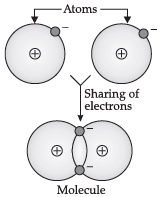(a) Why are covalent compounds generally poor conductors of electricity ?
(b) Name the following compound: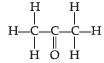(a) It is because they do not form ions.

(b) Propanone (Acetone)

Q.2. The elements of the third period of the Periodic table are given below: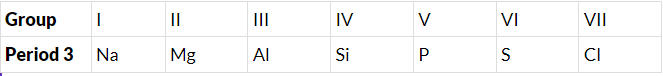(a) Which atom is bigger, Na or Mg? Why?
(b) Identify the most (i) metallic and (ii) non-metallic element in Period 3.

(a) Sodium is bigger than magnesium as it has lesser nuclear charge. So, there is less force of attraction between nucleus and valence electrons and less effective nuclear charge. It is, therefore, bigger in size.

(b) (i) Sodium is the most metallic element in period 3, as it can lose electrons easily due to its larger atomic size.
(ii) Chlorine is the most non-metallic element in period 3, because, it can gain electrons easily due to its smallest atomic size.

Q.3. In an experiment, a worm found in freshwater and slow-moving streams was cut into three pieces. It was observed that in due course of time, each cut piece of the worm develops to become a complete worm by growing all the missing parts.
(a) Identify the worm. Name another organism, which possesses the same characteristic of growing fully from its cut body parts.
(b) What is the name given to this type of reproduction? State whether the given organisms are unicellular and / or multicellular organisms.

(a) The worm is Planaria.

An example of other organism is "Hydra".
(b) The process is known as regeneration.

The given organisms are multicellular organism.

Q.4. Contraception is defined as the intentional prevention of conception through the use of various devices, sexual practices, chemicals, drugs, or surgical procedures. Thus, any device or act whose purpose is to prevent a woman from becoming pregnant can be considered as a contraceptive.
(a) Name any two contraceptive methods practiced only by women.
(b) How these contraceptive methods work?

(a) Oral pills and Loop/Copper-T are used by women only.

(b) (iOral pills change hormonal balance, so eggs are not released.

(ii) Loop/Copper-T are placed in the uterus. It prevents pregnancy by checking the entry of sperms through the vagina.

Q.5. In a cross between plants with homozygous pink flowers and plants with homozygous white flowers, all the off springs of F1 generation had pink flowers. When the F1 generation was self-crossed, it was observed in the F2 generation that out of 100, 75 flowers were pink. Make a cross and answer the following questions:
(a) What are the genotypes of the F2 progeny?
(b) What is the ratio of Pink: White flowers in the F2 generation?
OR
‘Gene control traits’? Explain this statement with an example.

(a) Genotypes of F2 progeny are PP, Pp and pp.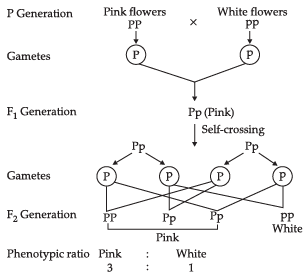(b) Ratio of pink and white flowers is: 3:1
OR
Genes are sections or segments of DNA that are carried on the chromosomes and determine specific human characteristics, such as height or eye colour. A gene codes for the formation of a particular protein which in turn controls the characteristics or traits.
For example: In pea plant, gene T is responsible for tallness of the plant. It gives instruction to the plant to synthesize a particular plant hormone. The amount of the plant hormone made will depend on the efficiency of the enzymes that make it. Consider now an enzyme that is important for this process. If this enzyme works efficiently, huge amount of hormone will be made, and the plant will be tall. If the gene for that enzyme has an alteration that makes the enzyme less efficient, the amount of hormone will be less, and the plant will be short. Thus genes control the traits.

Q.6. Garima Saini, performed an activity in school laboratory to observe the effects of magnetic field lines. Based on her observation, she has drawn the magnetic field lines of two magnets as shown in fig. A and fig. B.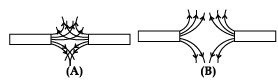(a) Select the figure that represents the correct pattern of field lines. Give reasons for your answer.
(b) Also, name the poles of the magnets facing each other.

OR
Identify the poles of the magnet in the given figures:
(a) Figure (1)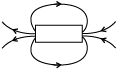(b) Figure (2)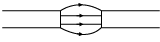(a) Figure B represents the correct pattern of field lines. In figure A, field lines cross each other which is not possible because if they cross each other, at the point of intersection, there would be two directions of field lines.
(b) In figure B, field lines are emerging in nature, so poles of magnet facing each other are north poles while opposite faces will have south polarity.
OR
(a) Field lines emerge from north pole (N) and merge at the south pole (S) in figure (1), So the poles of the magnet are: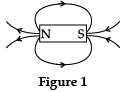(b) Field lines emerge from north pole (N) and merge at the south pole (S) in figure (2), So the poles of the magnet are: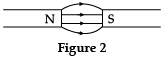Q.7. Let the amount of energy at fourth trophic level in the given food chain is 5 kJ, what will be the energy available at the producer level? Why do producers always occupy the first trophic level on every food chain?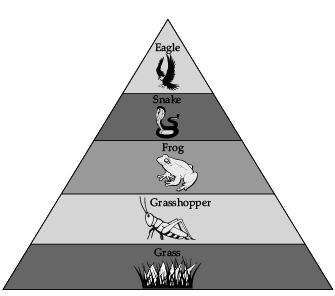OR
The following organisms form a food chain.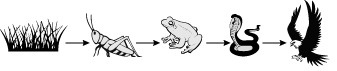Which of these will have the highest concentration of non-biodegradable chemicals? Name the phenomenon associated with it.

In the given food chain, if the amount of energy at fourth trophic level is 5 kJ, then 5000 kJ will be the energy available at the producer level. It is because, according to 10% law, only 10% of energy is transferred to the next trophic level and remaining 90% energy is used in life processes by present trophic level.
Producers always occupy the first trophic level on every food chain because producers (plants) have the ability to trap solar energy with the help of chlorophyll.
OR
Hawk will have the highest concentration of non-biodegradable chemicals.
The phenomenon associated with it is called biomagnification.

Section - B

Q.8. Study the organic compound given in the box. Answer the following questions.
Ethane , Ethene, Ethanoic acid, Ethyne, Ethanol
(a) The compound having functional groups—OH and –COOH.
(b) Gas used in welding.
(c) Homologue of the homologous series with general formula CnH2n+2.

(a)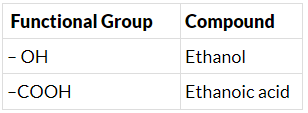(b) Ethyne
(c) Alkane : CnH2n+2
Ethane : C2H6

Q.9. Three elements ‘X’, ‘Y’ and ‘Z’ have atomic numbers 7, 8 and 9 respectively.
(a) Identify their positions (Group number and period number both) in the Modern Periodic Table.
(b) Arrange these elements in the decreasing order of their atomic radii.
(c) Write the formula of the compound formed when ‘X’ combines with ‘Z’.
OR
Study the data of the following three categories A, B and C of some elements.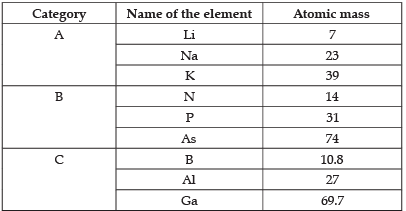(a) From the given three categories A, B and C, pick the one which forms Dobereiner's Triads.
(b) Why did Mendeleev placed elements of category A, B and C in three different groups?
(c) Is Newland's law of Octaves applicable to all the three categories?

(a) X (7) – 2, 5. Group 15; Period 2

Y (8 ) – 2, 6. Group 16; Period 2

Z (9) – 2, 7. Group 17; Period 2

(b) X > Y > Z
(c) XZ3
(a) X = Nitrogen
Y= Oxygen
Z = Fluorine

Groups:

• Nitrogen:  Group 15
• Oxygen: Group 16
• Fluorine: Group 17

Period: 2
(b)
Across a period, atomic radius decreases from left to right in the modern periodic table. Arrangement of element according to atomic radius in decreasing order:
Nitrogen > Oxygen > Fluorine

(c) When X combines with Z, compound formed is XZ3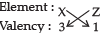Compound : XZ3
Thus, Compound formed is XZ3
OR
(a) Category A (Li, Na, K) forms Dobereiner ’s triads.
(b) Mendeleev arranged the elements in increasing order of atomic mass and grouped them as per similar chemical property. The elements in A, B and C are similar among themselves but have different chemical properties.
(c) No, Newland's law of Octaves is not applicable as it states that the chemical elements are arranged according to increasing atomic masses, those with similar physical and chemical properties occur after each interval of the eighth element.

Q.10. In one of his experiments with pea plants, Mendel observed that when a pure tall pea plant is crossed with a pure dwarf pea plant, in the first generation F1, only tall plants appear. What happens to the traits of the dwarf plants in this case? When the F1 generation plants were self-fertilised, he observed that, in the plants of second generation F2, both tall plants and dwarf plants were present. Why it happened? Explain briefly.

The dwarf traits of the plants is not expressed in the presence of the dominant tall trait.
In the Fgeneration, both the tall and dwarf traits are present in the ratio of 3:1, respectively. This showed that the traits for tallness and dwarfness are present in the F1 generation, but the dwarfness, being the recessive trait does not express itself in the presence of tallness i.e., the dominant trait.

Q.11. Two coils of resistance R1 = 3W and R= 9Ω are connected in series across a battery of potential difference 14 V.
(a) Draw the circuit diagram.
(b) Find the electrical energy consumed in 1 min in each resistance.

(a) The circuit diagram: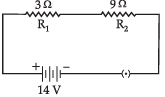(b) Given: R1 = 3 Ω
R2 = 9 Ω
In series connections
RS = R1 + R2
= 9 + 3 = 12 Ω
Now, I = V/R = 14/12 = 1.167A
Electric energy consumed in R1
H= I2R1t = (1.167)2 × 3 × 60 = 245.14J
Electric energy consumed in R2
H2 = I2R2t = (1.167)2 × 9 × 60 = 735.42J

Q.12. Find the current drawn from the battery by the network of four resistors shown in the figure.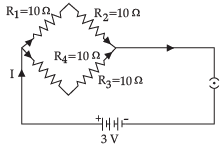OR
For the electric circuit given below, calculate: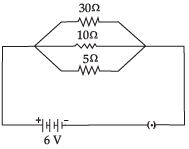(a) Current in each resistor.
(b) Total current drawn from the battery and
(c) Equivalent resistance of the circuit.

Given, V = 3 V
R1 = R2 = R3 = R4 = 10 Ω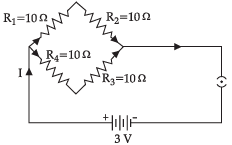In the given circuit, Rand R2 are in series. R3 and R4 are also in series connection
R5 = R1 + R2 = 10 + 10 = 20 Ω
R6 = R3 + R4 = 10 + 10 = 20 Ω
Now, R5 and R6 are in parallel connection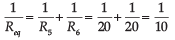⇒ Req = 10 Ω
By Ohm's law, V = IR
⇒ I = V/R = 3/10 = 0.3A
OR
(a) From Ohm's law,
V = IR ⇒ I = V/R
For 30Ω,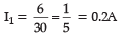For 10Ω, I2 = 6/10 = 0.6A
For 5Ω, I3 = 6/5 = 1.2 A
(b) In parallel connection,
Ieq = I1 +I2 + I3
= 0.2 + 0.6 + 1.2
Ieq = 2A
(c) All resistances are in parallel connection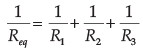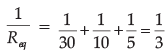⇒ Req = 3Ω

Q.13. While teaching the chapter "Our Environment" the teacher stressed upon the harmful effects of burning of fossil fuels, plastic paper, etc. The students noticed the extensive use of plastic and polythene in daily life can be avoided and the surroundings can be kept clean. They decided to make their school "Plastic and Polythene" free and motivated each other for its minimum use.
(a) Why should the use of polythene and plastic be reduced in daily life?
(b) In what way the students would have avoided the use of plastic and polythene in their school?

(a) Because they are non-biodegradable. Plastic and polythene cannot be degraded by the activity of microorganisms.
(b) (i) Carrying tiffin and water in steel containers.
(ii) Encourage the use of ink pens instead plastic pens. (ball pens are made up of plastic)/or any other.

Section - C

This section has 02 case-based questions (14 and 15). Each case is followed by 03 sub-questions (a), (b), (c). Parts (a) and (b) are compulsory. However, an internal choice has been provided in part (c).
Q.14. Sahil performed an experiment to study the inheritance pattern of genes. He crossed tall pea plants (TT) with short pea plants (tt) and obtained all tall plants in F1 generation.
(a) What set of genes will be present in the F1 generation?
(b) Give reason, why only tall plants are observed in F1 progeny.
(c) When F1 plants were self - pollinated, a total of 800 plants were produced. How many of these would be tall, medium height or short plants? Give the genotype of F2 generation.
OR
When F1 plants were cross-pollinated with plants having tt genes, a total of 800 plants were produced. How many of these would be tall, medium, or short plants? Give the genotype of F2 generation.

(a) Tt
(b) Both the recessive and dominant trait are passed on in F1 progeny. Out of the two trait only one of them is able to express itself in the progeny, which is called the dominant (T), while the other one is called the recessive (t). Here, the ‘tall’ trait is the dominant one.
(c) Out of 800 plants, 600 plants will be tall and 200 plants will be small.
1:2:1 (TT:Tt:tt)
OR
In the cross between Tt X tt, 400 Tall (Tt) and 400 short (tt) plants will be produced.
1:1(Tt:tt)

Q.15. Ansari Sir was demonstrating an experiment in his class with the setup as shown in the figure below.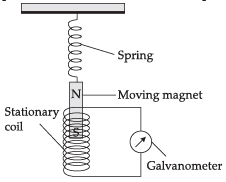A magnet is attached to a spring. The magnet can go in and out of the stationary coil.
He lifted the magnet and released it to make it oscillate through the coil. Based on your understanding of the phenomenon, answer the following questions.
(a) What is the principle which Ansari Sir is trying to demonstrate?
(b) What will be observed when the magnet starts oscillating through the coil. Explain the reason behind this observation.
(c) Consider the situation where the magnet goes in and out of the coil. State two changes which could be made to increase the deflection in the galvanometer.
OR
Is there any difference in the observations in the galvanometer when the magnet swings in and then out of the stationary coil? Justify your answer.

(a) Sir is trying to demonstrate the principle of Electromagnetic induction.
(b) There will be fluctuating induced current in the coil due to relative motion between the magnet and the coil. Accordingly the galvanometer needle will fluctuate on either side of 0 mark. Changing the magnetic field around the coil generates induced current.
(c) Using a stronger magnet, or using a coil with more number of turns.
OR
When the magnet moves into the coil, the galvanometer shows a momentary deflection towards one side, say left.
When the magnet moves out of the coil, the galvanometer shows a momentary deflection now towards right.
This is due to changing magnetic field /flux associated with the coil as the magnet moves in and out.
Alternatively, the flux increases when the magnet goes in and it decreases when the magnet goes out.

The document Class 10 Science: CBSE Sample Question Paper- Term II (2021-22) - 1 Notes | Study CBSE Sample Papers For Class 10 - Class 10 is a part of the Class 10 Course CBSE Sample Papers For Class 10.
All you need of Class 10 at this link: Class 10Use Code STAYHOME200 and get INR 200 additional OFF

## CBSE Sample Papers For Class 10

174 docs|7 tests

Track your progress, build streaks, highlight & save important lessons and more!

,

,

,

,

,

,

,

,

,

,

,

,

,

,

,

,

,

,

,

,

,

;